Courses

# Wave Guides (Part - 1) Electrical Engineering (EE) Notes | EduRev

## Electromagnetic Theory

Created by: Machine Experts

## Electrical Engineering (EE) : Wave Guides (Part - 1) Electrical Engineering (EE) Notes | EduRev

The document Wave Guides (Part - 1) Electrical Engineering (EE) Notes | EduRev is a part of the Electrical Engineering (EE) Course Electromagnetic Theory.
All you need of Electrical Engineering (EE) at this link: Electrical Engineering (EE)

Wave Guides

Electromagnetic waves carry both energy and momentum. It should therefore be possible to transmit energy contained in the wave from one place to another. For low frequencies (typically, less than 1 MHz), this is done by parallel transmission lines or coaxial cables. However, for higher frequencies, such as microwave frequencies, we need special conduits such a hollow metal tubes or optical fibers.

We will first explain the basic concepts of guiding waves by taking  the simple case of a pair of  parallel, infinite metal plates with a separation d between them. The wave is made to propagate in the hollow region between the plates, which we take to be empty space. We have seen that the electric field is primarily confined to the surface, penetrating a small “skin depth” which  becomes smaller with increasing frequency.  As a result we take the electric field to vanish at the surface of the guide.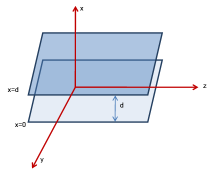Let us rewrite the Maxwell’s equations for the case where there are free charges or currents.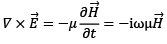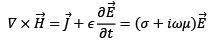where we have time variation to be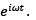Taking the curl of the these equations and substituting from the other equations, we get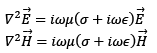For propagation between the plannes, σ = 0, so that we have,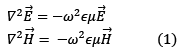Consider the original curl equations and write them in component form (with σ = 0)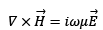gives,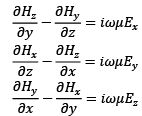Parallely, the derivatives of electric field components satisf,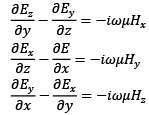The solutions of these set of equations can be classified into three distinct groups. The direction of propagation being along the z direction, we term this direction as  the longitudinal direction and a direction perpendicular to it (i.e. x and y directions) as the transverse direction.  The distinct solutions are grouped as

1. Solutions for which Ez = 0 , i.e. the non-zero electric field is transverse to the direction of propagation. This is called the "Transverse Electric " or TE mode. In this case the longitudinal component of magnetic field is non-vanishing (Hz≠0). The solution, therefore, is also referred to as H-mode.
2. Solutions for which Hz = 0 , i.e. the non-zero magnetic field is transverse to the direction of propagation. This is called the "Transverse Magnetic " or TM mode. In this case the longitudinal component of electric field is non-vanishing (Ez≠ 0). The solution, therefore, is also referred to as E-mode.
3. In some situations, it is possible to have the longitudinal component of both electric and magnetic field to be simultaneously zero, like the case of propagation of electromagnetic wave in free space. This special solution is called "Transverse Electric and Magnetic Mode" or TEM mode.

It is of course possible for a solution not to belong to any of these distinct categories in which case it would be a "mixed mode" solution.

Since the wave propagates along the z direction, the z dependence of the field is specified,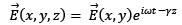where, the complex factor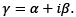Thus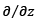is equivalent to multiplication by -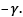Further, since the plates are of infinite extent in y direction, there is no field variation in this direction so that we can replace the derivativeby zero. Using these, we can rewrite the equations above as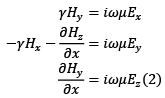and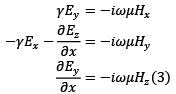The wave equation (1) takes the form,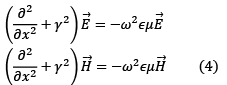We will discuss in detail the TE solution and leave the TM solution as an exercise.

TE- Mode :

In this case E= 0. From Eqn. (2) , we get Hy = constant, which we can choose to be zero. This in turn implies, from Eqn. (3), Ex = 0. We will first solve for Eusing Eqn. (4).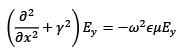Define k= ŷ2 + ω2∈μ. We have,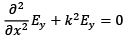the solution of which are well known to be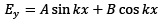(complete solution will be obtained by multiplying this with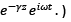We now insert the boundary condition, Ey = 0 on both the plates, i.e. at x= 0 and at x= d.

The former gives B= 0, so that Ey = A sin Kx. The latter condition restricts the values that k can take to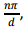where n = 1,2, … .( n cannot take the value zero because that would make the field identically zero. )

Thus we have,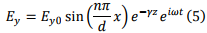where A = Ey0 is the maximum value of the field. We can now use this expression in Eqn. (3) to obtain the magnetic field components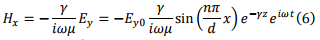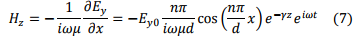Modes are named by specifying the value that n takes. As we have seen the lowest mode is n = 1. This is termed as TE10 mode, the meaning of the second index will be clear when we discuss rectangular waveguides but for the present case it remains zero for all values of n. The following figure gives the electric field profile for n=1 and n=2 for a fixed z.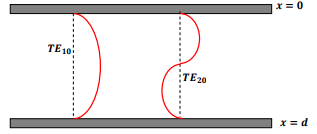If we look along the direction of propagation, for the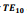mode, thefield lines crowdat the centre of the guide, where the field strength is strongest.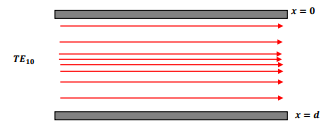The corresponding magnetic fields forare shown below: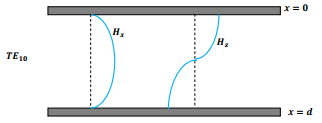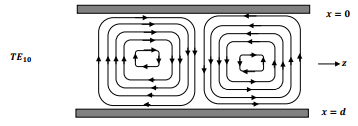Cutoff Frequency

Is transmission in this manner always possible? We have,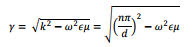Propagating solution implies that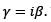Thus we requires,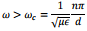If the frequency is less than this, the wave attenuates. The phase velocity for the propagating solution is given by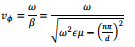As frequency decreases and approaches the critical value, it becomes infinite. For very large frequencies, the velocity in vacuum approaches that of light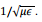TM Mode

We will not work out the TM mode algebra. In this case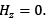. The non-zero field components are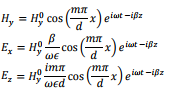TEM Mode

Note that in TM case , unlike in the case of TE modes, we can have m=0 here because the solutions are in terms of cosine functions. In this case we have,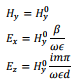which gives the ratio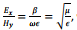which is the intrinsic impedance we have seen to characterize propagation of wave in a uniform medium.

Tutorial Assignment

1. For a guided wave between two infinite conducting planes separated by a distance of 0.25 m, find the cutoff frequency for the TM20 mode. If the operating frequency is 3 GHz, find the phase velocity of the wave.
2. A TE10 mode propagates between two parallel planes separated by a distance of 0.25 m. The planes are lossy and have a conductivity of 5 × 107 S/m. If the maximum electric field strength between the planes is 1000 V/m, determine the power loss per square meter on each plate when the operating frequency is 2 GHz.

Solutions to Tutorial Assignments

1. The critical angular frequencyωc is given by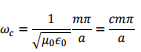so that the cutoff frequency is given by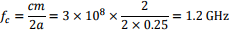If the operating frequency is 3 GHz, the propagation constant is given by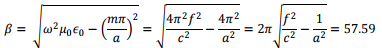The phase velocity is given by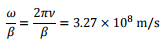2. The amplitude of linear current density on the plates is equal to the tangential component of the magnetic field on the planes,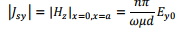Power loss per unit length of conductor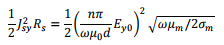We take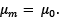. The loss for TE10 is (in J/m)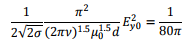Self Assessment Questions

1. For a guided wave between two infinite conducting planes separated by a distance of 0.2m. If the operating frequency is 3.3 GHz, find the number of distinct modes that can travel in the guide.
2. A TE20 mode is propagating along the z direction between two parallel conducting planes separated by 0.2m along the x- direction. Find the cutoff frequency. Determine λx. If the operating frequency is 2.5 GHz, determine λz and the non-vanishing components of the electric and the magnetic field. If the operating frequency is 1.2 GHz, calculate the distance over which the strength of the fields reduce to 1/e of their value.
3. Calculate the rate at which energy is transmitted in a parallel plane waveguide operating in TE10 mode

Solutions to Self Assessment Questions

1. The propagation constant is given by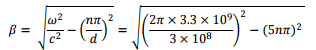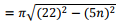For propagation to take place 22 > 5n, so that n<5. This implies 4 TE modes, 4 TM modes and one TEM mode, giving a total of 9 modes.

2. The cutoff frequency is given by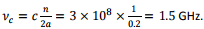The wavelength in the x direction for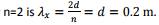m. If the operating frequency is 2.5 ×      109Hz., the propagation vector is given by (using n=2)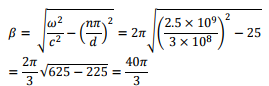The fields are as follows :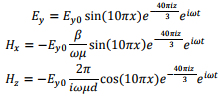To determine λz, we use the fact that it is equal to the distance over which the phase of the propagating wave changes by 2π. Thus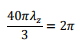so that λz= 3/20m.

If the operating frequency is 1.2 GHz, the wave attenuates, and we have,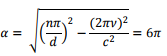so that the attenuation distance is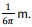3. The fields are given by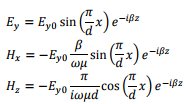Power transmitted per unit area is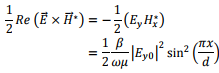Power transmitted in z direction through an area of unit width,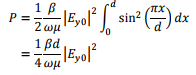,

,

,

,

,

,

,

,

,

,

,

,

,

,

,

,

,

,

,

,

,

;